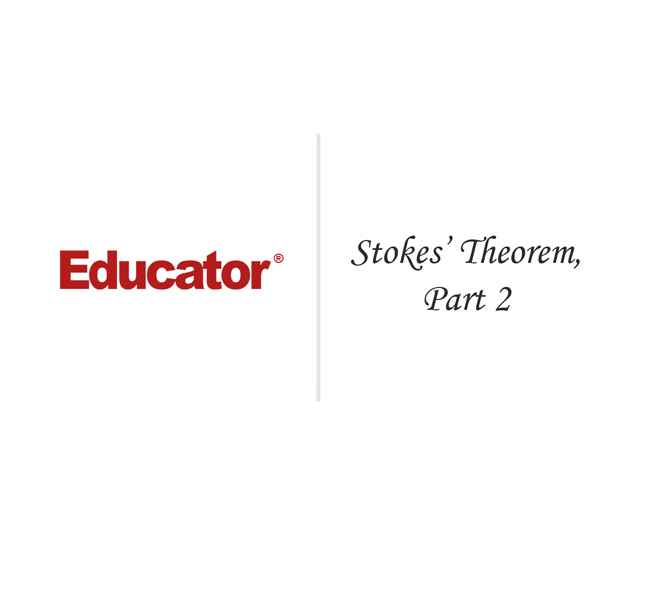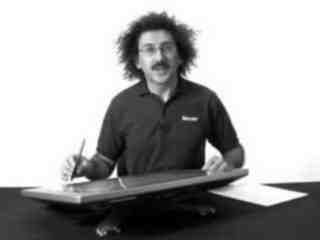Raffi Hovasapian

Stokes' Theorem, Part 2

Slide Duration:

Section 1: Vectors
Points & Vectors

28m 23s

Intro
0:00
Points and Vectors
1:02
A Point in a Plane
1:03
A Point in Space
3:14
Notation for a Space of a Given Space
6:34
Introduction to Vectors
9:51
14:51
Example 1
16:52
18:24
Example 2
21:01
Two More Properties of Vector Addition
24:16
Multiplication of a Vector by a Constant
25:27
Scalar Product & Norm

30m 25s

Intro
0:00
Scalar Product and Norm
1:05
Introduction to Scalar Product
1:06
Example 1
3:21
Properties of Scalar Product
6:14
Definition: Orthogonal
11:41
Example 2: Orthogonal
14:19
Definition: Norm of a Vector
15:30
Example 3
19:37
Distance Between Two Vectors
22:05
Example 4
27:19
More on Vectors & Norms

38m 18s

Intro
0:00
More on Vectors and Norms
0:38
Open Disc
0:39
Close Disc
3:14
Open Ball, Closed Ball, and the Sphere
5:22
Property and Definition of Unit Vector
7:16
Example 1
14:04
Three Special Unit Vectors
17:24
General Pythagorean Theorem
19:44
Projection
23:00
Example 2
28:35
Example 3
35:54
Inequalities & Parametric Lines

33m 19s

Intro
0:00
Inequalities and Parametric Lines
0:30
Starting Example
0:31
Theorem 1
5:10
Theorem 2
7:22
Definition 1: Parametric Equation of a Straight Line
10:16
Definition 2
17:38
Example 1
21:19
Example 2
25:20
Planes

29m 59s

Intro
0:00
Planes
0:18
Definition 1
0:19
Example 1
7:04
Example 2
12:45
General Definitions and Properties: 2 Vectors are Said to Be Paralleled If
14:50
Example 3
16:44
Example 4
20:17
More on Planes

34m 18s

Intro
0:00
More on Planes
0:25
Example 1
0:26
Distance From Some Point in Space to a Given Plane: Derivation
10:12
Final Formula for Distance
21:20
Example 2
23:09
Example 3: Part 1
26:56
Example 3: Part 2
31:46
Section 2: Differentiation of Vectors
Maps, Curves & Parameterizations

29m 48s

Intro
0:00
Maps, Curves and Parameterizations
1:10
Recall
1:11
Looking at y = x2 or f(x) = x2
2:23
Departure Space & Arrival Space
7:01
Looking at a 'Function' from ℝ to ℝ2
10:36
Example 1
14:50
Definition 1: Parameterized Curve
17:33
Example 2
21:56
Example 3
25:16
Differentiation of Vectors

39m 40s

Intro
0:00
Differentiation of Vectors
0:18
Example 1
0:19
Definition 1: Velocity of a Curve
1:45
Line Tangent to a Curve
6:10
Example 2
7:40
Definition 2: Speed of a Curve
12:18
Example 3
13:53
Definition 3: Acceleration Vector
16:37
Two Definitions for the Scalar Part of Acceleration
17:22
Rules for Differentiating Vectors: 1
19:52
Rules for Differentiating Vectors: 2
21:28
Rules for Differentiating Vectors: 3
22:03
Rules for Differentiating Vectors: 4
24:14
Example 4
26:57
Section 3: Functions of Several Variables
Functions of Several Variable

29m 31s

Intro
0:00
Length of a Curve in Space
0:25
Definition 1: Length of a Curve in Space
0:26
Extended Form
2:06
Example 1
3:40
Example 2
6:28
Functions of Several Variable
8:55
Functions of Several Variable
8:56
General Examples
11:11
Graph by Plotting
13:00
Example 1
16:31
Definition 1
18:33
Example 2
22:15
Equipotential Surfaces
25:27
Isothermal Surfaces
27:30
Partial Derivatives

23m 31s

Intro
0:00
Partial Derivatives
0:19
Example 1
0:20
Example 2
5:30
Example 3
7:48
Example 4
9:19
Definition 1
12:19
Example 5
14:24
Example 6
16:14
20:26
Higher and Mixed Partial Derivatives

30m 48s

Intro
0:00
Higher and Mixed Partial Derivatives
0:45
Definition 1: Open Set
0:46
Notation: Partial Derivatives
5:39
Example 1
12:00
Theorem 1
14:25
Now Consider a Function of Three Variables
16:50
Example 2
20:09
Caution
23:16
Example 3
25:42
Section 4: Chain Rule and The Gradient
The Chain Rule

28m 3s

Intro
0:00
The Chain Rule
0:45
Conceptual Example
0:46
Example 1
5:10
The Chain Rule
10:11
Example 2: Part 1
19:06
Example 2: Part 2 - Solving Directly
25:26
Tangent Plane

42m 25s

Intro
0:00
Tangent Plane
1:02
Tangent Plane Part 1
1:03
Tangent Plane Part 2
10:00
Tangent Plane Part 3
18:18
Tangent Plane Part 4
21:18
Definition 1: Tangent Plane to a Surface
27:46
Example 1: Find the Equation of the Plane Tangent to the Surface
31:18
Example 2: Find the Tangent Line to the Curve
36:54
Further Examples with Gradients & Tangents

47m 11s

Intro
0:00
Example 1: Parametric Equation for the Line Tangent to the Curve of Two Intersecting Surfaces
0:41
Part 1: Question
0:42
Part 2: When Two Surfaces in ℝ3 Intersect
4:31
Part 3: Diagrams
7:36
Part 4: Solution
12:10
Part 5: Diagram of Final Answer
23:52
Example 2: Gradients & Composite Functions
26:42
Part 1: Question
26:43
Part 2: Solution
29:21
Example 3: Cos of the Angle Between the Surfaces
39:20
Part 1: Question
39:21
Part 2: Definition of Angle Between Two Surfaces
41:04
Part 3: Solution
42:39
Directional Derivative

41m 22s

Intro
0:00
Directional Derivative
0:10
Rate of Change & Direction Overview
0:11
Rate of Change : Function of Two Variables
4:32
Directional Derivative
10:13
Example 1
18:26
Examining Gradient of f(p) ∙ A When A is a Unit Vector
25:30
Directional Derivative of f(p)
31:03
33:23
Example 2
34:53
A Unified View of Derivatives for Mappings

39m 41s

Intro
0:00
A Unified View of Derivatives for Mappings
1:29
Derivatives for Mappings
1:30
Example 1
5:46
Example 2
8:25
Example 3
12:08
Example 4
14:35
Derivative for Mappings of Composite Function
17:47
Example 5
22:15
Example 6
28:42
Section 5: Maxima and Minima
Maxima & Minima

36m 41s

Intro
0:00
Maxima and Minima
0:35
Definition 1: Critical Point
0:36
Example 1: Find the Critical Values
2:48
Definition 2: Local Max & Local Min
10:03
Theorem 1
14:10
Example 2: Local Max, Min, and Extreme
18:28
Definition 3: Boundary Point
27:00
Definition 4: Closed Set
29:50
Definition 5: Bounded Set
31:32
Theorem 2
33:34
Further Examples with Extrema

32m 48s

Intro
0:00
Further Example with Extrema
1:02
Example 1: Max and Min Values of f on the Square
1:03
Example 2: Find the Extreme for f(x,y) = x² + 2y² - x
10:44
Example 3: Max and Min Value of f(x,y) = (x²+ y²)⁻¹ in the Region (x -2)²+ y² ≤ 1
17:20
Lagrange Multipliers

32m 32s

Intro
0:00
Lagrange Multipliers
1:13
Theorem 1
1:14
Method
6:35
Example 1: Find the Largest and Smallest Values that f Achieves Subject to g
9:14
Example 2: Find the Max & Min Values of f(x,y)= 3x + 4y on the Circle x² + y² = 1
22:18
More Lagrange Multiplier Examples

27m 42s

Intro
0:00
Example 1: Find the Point on the Surface z² -xy = 1 Closet to the Origin
0:54
Part 1
0:55
Part 2
7:37
Part 3
10:44
Example 2: Find the Max & Min of f(x,y) = x² + 2y - x on the Closed Disc of Radius 1 Centered at the Origin
16:05
Part 1
16:06
Part 2
19:33
Part 3
23:17
Lagrange Multipliers, Continued

31m 47s

Intro
0:00
Lagrange Multipliers
0:42
First Example of Lesson 20
0:44
Let's Look at This Geometrically
3:12
Example 1: Lagrange Multiplier Problem with 2 Constraints
8:42
Part 1: Question
8:43
Part 2: What We Have to Solve
15:13
Part 3: Case 1
20:49
Part 4: Case 2
22:59
Part 5: Final Solution
25:45
Section 6: Line Integrals and Potential Functions
Line Integrals

36m 8s

Intro
0:00
Line Integrals
0:18
Introduction to Line Integrals
0:19
Definition 1: Vector Field
3:57
Example 1
5:46
Example 2: Gradient Operator & Vector Field
8:06
Example 3
12:19
Vector Field, Curve in Space & Line Integrals
14:07
Definition 2: F(C(t)) ∙ C'(t) is a Function of t
17:45
Example 4
18:10
Definition 3: Line Integrals
20:21
Example 5
25:00
Example 6
30:33
More on Line Integrals

28m 4s

Intro
0:00
More on Line Integrals
0:10
Line Integrals Notation
0:11
Curve Given in Non-parameterized Way: In General
4:34
Curve Given in Non-parameterized Way: For the Circle of Radius r
6:07
Curve Given in Non-parameterized Way: For a Straight Line Segment Between P & Q
6:32
The Integral is Independent of the Parameterization Chosen
7:17
Example 1: Find the Integral on the Ellipse Centered at the Origin
9:18
Example 2: Find the Integral of the Vector Field
16:26
Discussion of Result and Vector Field for Example 2
23:52
Graphical Example
26:03
Line Integrals, Part 3

29m 30s

Intro
0:00
Line Integrals
0:12
Piecewise Continuous Path
0:13
Closed Path
1:47
Example 1: Find the Integral
3:50
The Reverse Path
14:14
Theorem 1
16:18
Parameterization for the Reverse Path
17:24
Example 2
18:50
Line Integrals of Functions on ℝn
21:36
Example 3
24:20
Potential Functions

40m 19s

Intro
0:00
Potential Functions
0:08
Definition 1: Potential Functions
0:09
Definition 2: An Open Set S is Called Connected if…
5:52
Theorem 1
8:19
Existence of a Potential Function
11:04
Theorem 2
18:06
Example 1
22:18
Contrapositive and Positive Form of the Theorem
28:02
The Converse is Not Generally True
30:59
Our Theorem
32:55
Compare the n-th Term Test for Divergence of an Infinite Series
36:00
So for Our Theorem
38:16
Potential Functions, Continued

31m 45s

Intro
0:00
Potential Functions
0:52
Theorem 1
0:53
Example 1
4:00
Theorem in 3-Space
14:07
Example 2
17:53
Example 3
24:07
Potential Functions, Conclusion & Summary

28m 22s

Intro
0:00
Potential Functions
0:16
Theorem 1
0:17
In Other Words
3:25
Corollary
5:22
Example 1
7:45
Theorem 2
11:34
Summary on Potential Functions 1
15:32
Summary on Potential Functions 2
17:26
Summary on Potential Functions 3
18:43
Case 1
19:24
Case 2
20:48
Case 3
21:35
Example 2
23:59
Section 7: Double Integrals
Double Integrals

29m 46s

Intro
0:00
Double Integrals
0:52
Introduction to Double Integrals
0:53
Function with Two Variables
3:39
Example 1: Find the Integral of xy³ over the Region x ϵ[1,2] & y ϵ[4,6]
9:42
Example 2: f(x,y) = x²y & R be the Region Such That x ϵ[2,3] & x² ≤ y ≤ x³
15:07
Example 3: f(x,y) = 4xy over the Region Bounded by y= 0, y= x, and y= -x+3
19:20
Polar Coordinates

36m 17s

Intro
0:00
Polar Coordinates
0:50
Polar Coordinates
0:51
Example 1: Let (x,y) = (6,√6), Convert to Polar Coordinates
3:24
Example 2: Express the Circle (x-2)² + y² = 4 in Polar Form.
5:46
Graphing Function in Polar Form.
10:02
Converting a Region in the xy-plane to Polar Coordinates
14:14
Example 3: Find the Integral over the Region Bounded by the Semicircle
20:06
Example 4: Find the Integral over the Region
27:57
Example 5: Find the Integral of f(x,y) = x² over the Region Contained by r= 1 - cosθ
32:55
Green's Theorem

38m 1s

Intro
0:00
Green's Theorem
0:38
Introduction to Green's Theorem and Notations
0:39
Green's Theorem
3:17
Example 1: Find the Integral of the Vector Field around the Ellipse
8:30
Verifying Green's Theorem with Example 1
15:35
A More General Version of Green's Theorem
20:03
Example 2
22:59
Example 3
26:30
Example 4
32:05
Divergence & Curl of a Vector Field

37m 16s

Intro
0:00
Divergence & Curl of a Vector Field
0:18
Definitions: Divergence(F) & Curl(F)
0:19
Example 1: Evaluate Divergence(F) and Curl(F)
3:43
Properties of Divergence
9:24
Properties of Curl
12:24
Two Versions of Green's Theorem: Circulation - Curl
17:46
Two Versions of Green's Theorem: Flux Divergence
19:09
Circulation-Curl Part 1
20:08
Circulation-Curl Part 2
28:29
Example 2
32:06
Divergence & Curl, Continued

33m 7s

Intro
0:00
Divergence & Curl, Continued
0:24
Divergence Part 1
0:25
Divergence Part 2: Right Normal Vector and Left Normal Vector
5:28
Divergence Part 3
9:09
Divergence Part 4
13:51
Divergence Part 5
19:19
Example 1
23:40
Final Comments on Divergence & Curl

16m 49s

Intro
0:00
Final Comments on Divergence and Curl
0:37
Several Symbolic Representations for Green's Theorem
0:38
Circulation-Curl
9:44
Flux Divergence
11:02
Closing Comments on Divergence and Curl
15:04
Section 8: Triple Integrals
Triple Integrals

27m 24s

Intro
0:00
Triple Integrals
0:21
Example 1
2:01
Example 2
9:42
Example 3
15:25
Example 4
20:54
Cylindrical & Spherical Coordinates

35m 33s

Intro
0:00
Cylindrical and Spherical Coordinates
0:42
Cylindrical Coordinates
0:43
When Integrating Over a Region in 3-space, Upon Transformation the Triple Integral Becomes..
4:29
Example 1
6:27
The Cartesian Integral
15:00
Introduction to Spherical Coordinates
19:44
Reason It's Called Spherical Coordinates
22:49
Spherical Transformation
26:12
Example 2
29:23
Section 9: Surface Integrals and Stokes' Theorem
Parameterizing Surfaces & Cross Product

41m 29s

Intro
0:00
Parameterizing Surfaces
0:40
Describing a Line or a Curve Parametrically
0:41
Describing a Line or a Curve Parametrically: Example
1:52
Describing a Surface Parametrically
2:58
Describing a Surface Parametrically: Example
5:30
Recall: Parameterizations are not Unique
7:18
Example 1: Sphere of Radius R
8:22
Example 2: Another P for the Sphere of Radius R
10:52
This is True in General
13:35
Example 3: Paraboloid
15:05
Example 4: A Surface of Revolution around z-axis
18:10
Cross Product
23:15
Defining Cross Product
23:16
Example 5: Part 1
28:04
Example 5: Part 2 - Right Hand Rule
32:31
Example 6
37:20
Tangent Plane & Normal Vector to a Surface

37m 6s

Intro
0:00
Tangent Plane and Normal Vector to a Surface
0:35
Tangent Plane and Normal Vector to a Surface Part 1
0:36
Tangent Plane and Normal Vector to a Surface Part 2
5:22
Tangent Plane and Normal Vector to a Surface Part 3
13:42
Example 1: Question & Solution
17:59
Example 1: Illustrative Explanation of the Solution
28:37
Example 2: Question & Solution
30:55
Example 2: Illustrative Explanation of the Solution
35:10
Surface Area

32m 48s

Intro
0:00
Surface Area
0:27
Introduction to Surface Area
0:28
Given a Surface in 3-space and a Parameterization P
3:31
Defining Surface Area
7:46
Curve Length
10:52
Example 1: Find the Are of a Sphere of Radius R
15:03
Example 2: Find the Area of the Paraboloid z= x² + y² for 0 ≤ z ≤ 5
19:10
Example 2: Writing the Answer in Polar Coordinates
28:07
Surface Integrals

46m 52s

Intro
0:00
Surface Integrals
0:25
Introduction to Surface Integrals
0:26
General Integral for Surface Are of Any Parameterization
3:03
Integral of a Function Over a Surface
4:47
Example 1
9:53
Integral of a Vector Field Over a Surface
17:20
Example 2
22:15
Side Note: Be Very Careful
28:58
Example 3
30:42
Summary
43:57
Divergence & Curl in 3-Space

23m 40s

Intro
0:00
Divergence and Curl in 3-Space
0:26
Introduction to Divergence and Curl in 3-Space
0:27
Define: Divergence of F
2:50
Define: Curl of F
4:12
The Del Operator
6:25
Symbolically: Div(F)
9:03
Symbolically: Curl(F)
10:50
Example 1
14:07
Example 2
18:01
Divergence Theorem in 3-Space

34m 12s

Intro
0:00
Divergence Theorem in 3-Space
0:36
Green's Flux-Divergence
0:37
Divergence Theorem in 3-Space
3:34
Note: Closed Surface
6:43
Figure: Paraboloid
8:44
Example 1
12:13
Example 2
18:50
Recap for Surfaces: Introduction
27:50
Recap for Surfaces: Surface Area
29:16
Recap for Surfaces: Surface Integral of a Function
29:50
Recap for Surfaces: Surface Integral of a Vector Field
30:39
Recap for Surfaces: Divergence Theorem
32:32
Stokes' Theorem, Part 1

22m 1s

Intro
0:00
Stokes' Theorem
0:25
Recall Circulation-Curl Version of Green's Theorem
0:26
Constructing a Surface in 3-Space
2:26
Stokes' Theorem
5:34
Note on Curve and Vector Field in 3-Space
9:50
Example 1: Find the Circulation of F around the Curve
12:40
Part 1: Question
12:48
Part 2: Drawing the Figure
13:56
Part 3: Solution
16:08
Stokes' Theorem, Part 2

20m 32s

Intro
0:00
Example 1: Calculate the Boundary of the Surface and the Circulation of F around this Boundary
0:30
Part 1: Question
0:31
Part 2: Drawing the Figure
2:02
Part 3: Solution
5:24
Example 2: Calculate the Boundary of the Surface and the Circulation of F around this Boundary
13:11
Part 1: Question
13:12
Part 2: Solution
13:56
Bookmark & Share Embed

## Copy & Paste this embed code into your website’s HTML

Please ensure that your website editor is in text mode when you paste the code.
(In Wordpress, the mode button is on the top right corner.)
×
• - Allow users to view the embedded video in full-size.
Since this lesson is not free, only the preview will appear on your website.

• ## Related Books1 answerLast reply by: Professor HovasapianMon Dec 21, 2015 7:28 PMPost by Hen McGibbons on December 16, 2015fantastic course. your ability to explain complex concepts in an understandable way is a powerful skill.as a freshman in college, i can only think of 2 teachers that have this same ability. one of them was an ap us history teacher and the other was an intro psychology professor. I believe that multivariable calculus is even more difficult to grasp than those subjects, so I give you even more credit!I'm curious, can you list all the subjects you have been trained to teach or have taught in the past? I know your expertise is not limited to mathematics because I saw that you have many chemistry videos.thanks,hen1 answerLast reply by: Professor HovasapianSat Aug 17, 2013 8:24 PMPost by Mikael Svedmyr on August 17, 2013Thank you so much for a great course. I now have an understanding for these matters that I did not achive during the course in my university. However, I was wondering if you plan to add new lessons about Taylor's series in multivarible calculus.Thank you again.1 answerLast reply by: Professor HovasapianMon Jul 8, 2013 4:08 PMPost by Josh Winfield on July 8, 2013Results are in! MATH201 (Multivariate And Vector Calculus) Mark 90 (High Distinction)Thanks so Much Raffi, not only for helping me to get a good grade but for helping me too fully understand the maths its applications and it power!Josh (Australia)1 answerLast reply by: Professor HovasapianWed Jul 3, 2013 9:56 PMPost by barry ben-ishai on June 29, 2013Great Job teaching :-)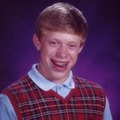1 answerLast reply by: Professor HovasapianSun Apr 21, 2013 4:14 AMPost by Riley Argue on April 18, 2013Thanks

### Stokes' Theorem, Part 2

Describe the boundary of the surface above the xy - plane and bounded by y2 + z2 = 1 with − 1 ≤ x ≤ 1.
• Note that y2 + z2 = 1 is a cylinder of radius 1 which goes along the x - axis.
• The xy - plane cuts this cylinder in half, and the restriction − 1 ≤ x ≤ 1 cuts the cylinder into a measureable section.
Hence the boundary of the surface is a square with vertices at ( − 1, − 1), ( − 1,1), (1,1) and (1, − 1).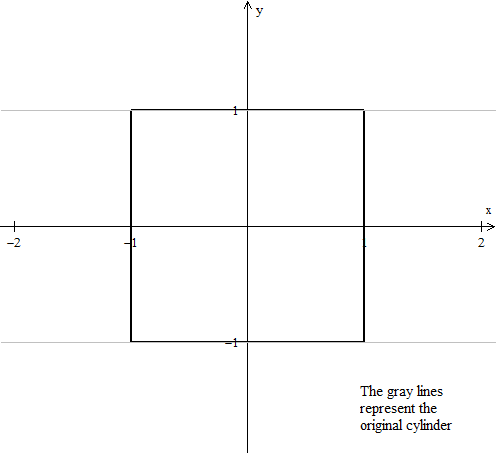Describe the boundary of the surface above the first octant and below the plane x + y + z = 5.
• Note that our region is a tetrahedron with vertices at the origin, (5,0,0), (0,5,0) and (0,0,5).
Our boundary is therefore a triangle.
The boundary below describes the surface S of a region. Determine which integral from Stoke's Theorem is most convenient for the region.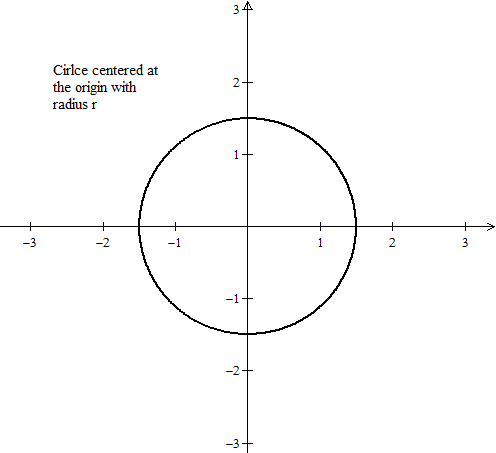Since we can parametrize a circle through C(t) = (cos(t),sin(t)), Stokes's theorem works best for the integral ∫B F(C(t)) ×C′(t) dt where B is the boundary.
The boundary below describes the surface S of a region. Determine which integral from Stoke's Theorem is most convenient for the region.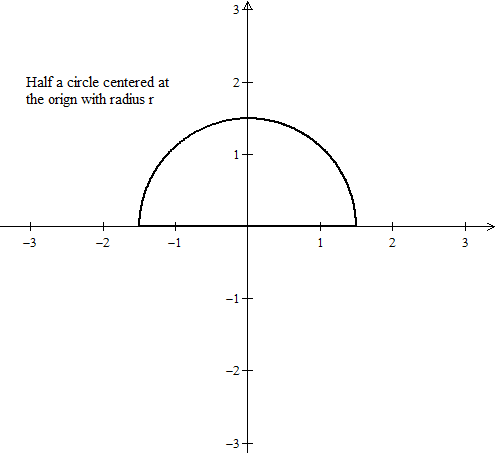• We can parametrize the circle through C(t) = (cos(t),sin(t)) as well as a line through x(t) = P + t(Q − P).
We would be adding two integrals, but Stokes's theorem works best for the integral ∫B F(C(t)) ×C′(t) dt where B is the boundary.
The boundary below describes the surface S of a region. Determine which integral from Stoke's Theorem is most convenient for the region.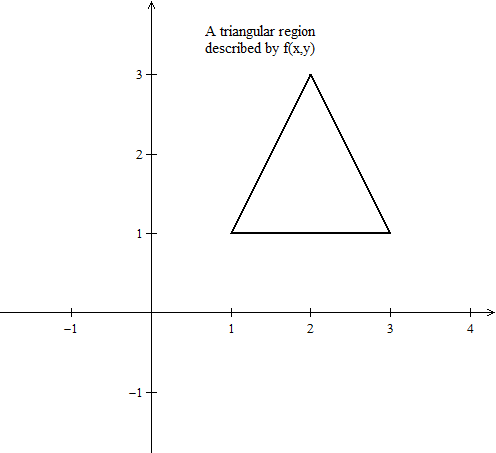• We can parametrize the three lines through x(t) = P + t(Q − P), but we would be adding three integrals.
Since we can parametrize f(x,y), Stokes's theorem works best for the double integral dt where S is the region the surface lays in.
The boundary below describes the surface S of a region. Determine which integral from Stoke's Theorem is most convenient for the region.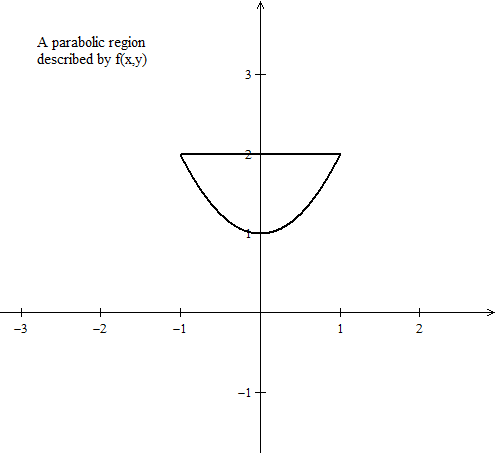• We can parametrize the parabola with C(t) = (t,t2) as well as a line through x(t) = P + t(Q − P).
We would be adding two integrals, but Stokes's theorem works best for the integral ∫B F(C(t)) ×C′(t) dt where B is the boundary.
The boundary below describes the surface S of a region. Determine which integral from Stoke's Theorem is most convenient for the region.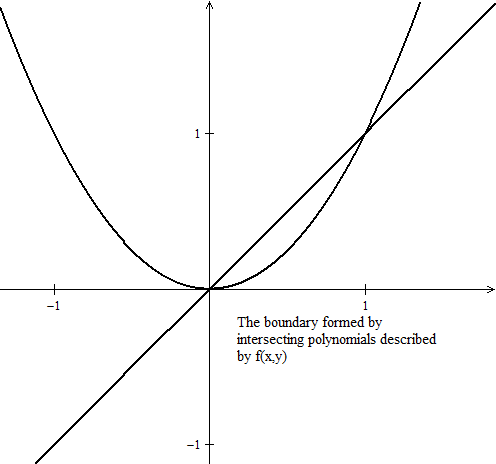• Although we may be able to compute two integrals (as well as represent the boundaries parametrically) it is easier to compute the double integration for the area.
Hence, Stokes's theorem works best for the double integral dt where S is the region the surface lays in.
The boundary below describes the surface S of a region. Determine which integral from Stoke's Theorem is most convenient for the region.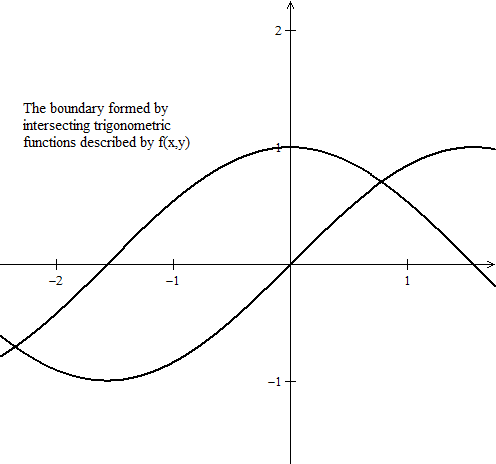In this scenario it is best to compute the double integration for the area, Stokes's theorem works best for the double integral dt where S is the region the surface lays in.
The boundary below describes the surface S of a region. Determine which integral from Stoke's Theorem is most convenient for the region.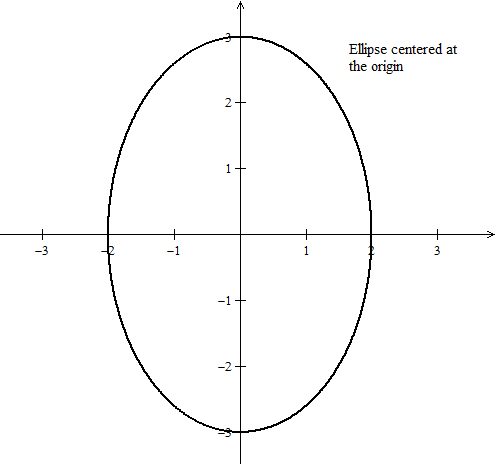• For ellipses, computing the double integral for the area may be tedious at first, but we can always use polar coordinates to simplify our calculations.
Hence, Stokes's theorem works best for the double integral dt where S is the region the surface lays in.
The boundary below describes the surface S of a region. Determine which integral from Stoke's Theorem is most convenient for the region.• In order for Stoke's Theorem to apply, our orientation must be counterclockwise.
• Recall that we can always change the orientation of a curve as ∫C F = − ∫C F . Hence our parametrization of our circle still applies as before.
Therefore, Stokes's theorem works best for the integral ∫B F(C(t)) ×C′(t) dt where B is the boundary.

*These practice questions are only helpful when you work on them offline on a piece of paper and then use the solution steps function to check your answer.

### Stokes' Theorem, Part 2

Lecture Slides are screen-captured images of important points in the lecture. Students can download and print out these lecture slide images to do practice problems as well as take notes while watching the lecture.

• Intro 0:00
• Example 1: Calculate the Boundary of the Surface and the Circulation of F around this Boundary 0:30
• Part 1: Question
• Part 2: Drawing the Figure
• Part 3: Solution
• Example 2: Calculate the Boundary of the Surface and the Circulation of F around this Boundary 13:11
• Part 1: Question
• Part 2: Solution

### Transcription: Stokes' Theorem, Part 2

Hello and welcome back to educator.com and multivariable calculus.0000

In the last lesson, we started talking about Stoke's Theorem, we discussed a little bit of its background. We did an example.0004

Today, we are going to continue on with some more examples of Stoke's Theorem.0008

Again, Stoke's Theorem is the one that seems to give kids the biggest problem, and yet interestingly enough, it is the one that generalizes most naturally.0013

It is just the circulation curl form of Green's Theorem kicked up one dimension. Let us just jump in and do some examples. Okay.0022

So, this time -- let us see here -- We will do example 1. This time we will define our surface first, so let s be the surface z = 4 - x2 - y2, and z > or = 0.0032

They give it to us in the form of an equation, we have to find out, we have to draw it out, find out what it is, and let f = z - yx + z - x -y.0061

Okay, in this particular case, we want you to find a couple of things. We want you to find the ds, which is the boundary, remember this symbol is the boundary of the surface, and calculate the circulation of f around this boundary.0085

Okay. Let us go ahead and draw out what this looks like. 4 - x2y2, this is a paraboloid that is inverted, and it starts up at 4.0121

When x is 0, y is 0, z = 4. So, what you have is basically something that goes down like this, goes down like this, and goes around like this.0138

That is it. It is a paraboloid that is just upside down. z > or = 0 means it is everything above the xy plane.0150

So, we have this surface right here, okay, so this is the surface. This is s. The boundary of this surface is this right here, this curve. It is the circle in the xy plane.0160

So, let us see here. Let us see how we can, so two, this is two, and it would be two this way, 4, so it looks like... so this is our boundary curve, and that is what is really, really important here.0180

So, imagine this is kind of like some hat, the bottom of the hat, the circle that the hat makes, that is the boundary of the surface.0202

Imagine if I took some circle, and if I took the piece of material that is in between that circle, and if I just deformed it, if I pulled it and stretched it, so now I have this tent like thing, right?0214

Well, the boundary itself has not changed. I have deformed the surface. I have not changed anything else, so as it turns out, this circle is the boundary of the flat region, or if it is the boundary of any deformation that I make to that flat region.0226

This is like a hat, that is the boundary. So, this -- oops -- Δs.0241

So, that is going to equal the following. It is of course a circle in the xy plane, so it is going to be a function of x and y.0251

It is going to be a circle, and let us see what its radius is going to be. Well, let us see.0261

If this is going to be... if this is 4 - x2 - y2, therefore the radius is going to have to be 2, so this is going to be x2 + y2 = 4, = 22, because the radius of this circle is 2.0266

I will write it this way. x2 + y2 = 22. That is the boundary curve, okay?0287

If I wanted to find the circulation, I could find the circulation of this vector field directly by integrating around this boundary curve, which I can parameterize by 2cos(Θ), 2sin(Θ), this is just a curve in the xy plane.0292

But I am not going to do that. I am going to go ahead and solve this via Stoke's Theorem.0310

We want to integrate over the surface, not the boundary of the surface. That is the whole idea. We are getting some practice with Stoke's theorem, but that is the boundary. I hope that makes sense. Okay.0314

So, let us use, let us see... so let us calculate over s the curl of the vector field of the parameterization dotted n dt du.0327

Let us actually calculate the flux of the curl vector field. That is what we are doing. The surface integral, in other words. Let us calculate the surface integral of the curl. Let us use Stoke's Theorem.0355

Well, let us go ahead and see what our... parameterization, surface... okay, so let us go ahead and write down actually, you know what, let us start on another page. So let us write down what our parameterization is, because we need a parameterization.0370

Well, they said that z is equal to 4 - x2 - y2, so they are giving us the surface as a function of x and y.0387

We know that our parameterization is going to equal x,y, and z itself, which is 4 - x2 - y2. That is our parameterization for our surface.0398

Well, we want to go ahead and form dp dx. dp dx is going to equal (1,0,-2x).0409

We want to form dp dy. That is going to equal (0,1,-2y).0419

We want to form n, which is equal to the cross product of these two, so that is going to equal, I am going to go ahead and write this one out actually, i, j, k... (1,0) - 2x (0,1) - 2y, and when I go ahead and remember cross product, we do a determinant, symbolic calculation.0430

When I do that, I get that n is actually equal to 2x, 2y, and 1. That is the normal vector to this surface. In other words, if I have this upside down parabola, at some point there is some vector sticking out at that point. That is what this is. It is the normal vector to this surface.0455

So, we have n. Okay. Now let us go ahead and find the curl of the vector field.0475

So the curl of f, that is also a symbolic determinant calculation. This time I am going to use capital D notation. Oops, d2, d3.0481

I have z - y, I have x + z, and I have -x - y. Okay, so at this point you should be reasonably comfortable with calculating... expanding determinants along the first row, and finding what the curl of a vector field is.0498

When I do this, I get the following. I get (-2,2,2). Okay.0515

Now, we want to find the following. We want to find the curl of f of the parameterization.0524

Well the curl of f of the parameterization, in this case the curl of f is a constant vector, so I do not have to do anything with the parameterization and the curl.0536

In the next example, I actually will do that. So, this is just (-2,2,2). Well, of course the next step is to find the curl of f of p dotted with n.0545

When I do that, so I do this, dot it with this and I end up with -4x + 4y + 2. That is going to be my integrand. Therefore my final integral looks like this.0562

Okay. Let us go ahead and draw out this region. Remember we said we had a circle, right? A circle of radius 2. So, this is x2 + y2 = 4.0583

That means y = 4 - x2 all under the radical sign + or -, and x... so x is going to go from -2 to 2.0601

y is going to go from -sqrt(4 - x2) all the way to +sqrt(4 - x2).0618

The curl of f(p) dotted with n is my integrand. That is -4x + 4y + 2, and this is y and this is x, so we do y first... x.0627

When I put this into my mathematical software, I get 8pi. That is it. Positive circulation, this thing is actually rotating. It is rotating in a counter-clockwise direction. That is what is happening, that is it. Nice and straight forward and simple.0644

Find the region, find the parameterization, in this particular case find the boundary and then you can decide if you are actually going to do the circulation integral, or if you are going to do the surface integral.0661

In this case we decided to do Stoke's Theorem, so we decided to integrate over the surface. We had the parameterization because we know what that is. We are given what the surface was. Then we just filled in the rest.0674

We found n, we found the curl of f, we found the curl of f of p, we took the dot product of the two things, and we figured out what the region was, -2 to 2, -sqrt(4-x2) to + sqrt(4-x2), and you just solve the integral.0687

Okay. So, little note here. Now, in this example and the example from the previous lesson, in the previous two examples... the curl of f was a constant vector.0706

So, we did not have to form, at least explicitly, this thing... the curl of f of p... we did not have to form the composite function, which is what the integrand requires.0746

The double integral, that is what is requires. Okay. Now, let us go ahead and do an example where we do have to do that. Where we do have to run through the entire process.0766

So, example number 2 here. I think I am going to do this one in red ink. So, example number 2.0781

We are going to let s be the same surface. Let s be the same surface as before, in other words the upside down paraboloid, be the same surface which is z = 4 - x2 - y2, z > or = 0.0800

This time we will let our vector field f, we will let it equal to xy, yz, and xz. Okay.0824

So, we have our parameterization, which is what we always want to start off with, xy is x and y and of course the function itself. 4 - x2 - y2.0838

Okay. We already know what n is, we calculated it from before, so let us go ahead and write down what that is.0851

So, n = (2x,2y,1).0856

So, now let us go ahead and calculate the curl of f. The curl of this particular vector field, well let us go ahead and form our symbolic determinant i,j,k d dx, d dy, and d dz.0867

We have xy, yz, and we have xz. When we expand this determinant along the first row, we get the following. We get (-y,-z,-x). So this is not a constant vector. Okay.0887

Now, we are going to form the curl of f(p), we are going to form the composite function. This is the curl of f, we are going to put the parameterization into that. The curl of f(p).0906

Wherever I see y, I put the second coordinate function. Wherever I see z, I put the first coordinate function, wherever I see x, I put the third coordinate function.0923

Okay... equals -y, okay, let us write down what the parameterization is so we remind ourselves -- I am going to do this one in blue, we have it on the same page -- the parameterization of xy is equal to x and y, 4 - x2 - y2.0934

So, -y, that just stays -y. -z, well -z is - this thing. This is z.0955

So, it is going to be x2 + y2 - 4 and of course, -x, that is -x, that stays the same... -x. this is our curl of f(p).0969

Now, we have to form the curl of f(p) dotted with n. Well, let us go ahead and remind ourselves what n is. N = (2x, 2y, 1).0985

We are going to take the dot product of this thing, and this thing. When we do that, here is what we get.1007

The dot product of this and this is this × that. So it is going to be -2xy, and it is going to be + this × that.1015

It is going to be... I am going to write it the way I have it here... -8y + 2x2y + 2y3, and then + this × that, - x.1026

There you go. This is our integrand, right here. Now, we are ready to solve the integral.1051

This one I am going to write out entirely. So, the integral over s of the curl of f(p) dotted with n dt du = -2 to 2, -sqrt(4 - x2) all the way to +sqrt(4 - x2), - 2xy - 8y + 2x2y + 2y3 - x, dy dx.1061

When I put that into my mathematical software, I get 0. That is it. In this particular case, this particular vector field is not this vector field.... xy, yz, xz, it is not rotating around that surface. It is not circulating around the boundary. That is what this says.1112

Very, very simple. I find n, I have p, I find the curl of f, I form the composite function, curl of f(p), that is a vector.1134

I am going to dot that vector with vector n. That gives me a scalar. That is this thing. That is my integrand. I take my integrand, I take a look at my domain for my parameter, in this case -2 to 2, this is this to this for y, and I solve it.1147

I get a particular number. That is all that is going on here. In case you lose your way, just work symbolically. As long as you have this, you can just run down the list and find what you need.1163

That is what is important. So, I hope you have an opportunity to practice this as much as possible.1176

Again, there is no one thing that you can always do over and over and over again. The process is the same, but the problem is going to come in different ways.1182

Sometimes you are given a parameterization, sometimes you are not. Sometimes you are... it is described one way, sometimes it is described another way.1193

Sometimes you will have to solve the line integral, sometimes you will have to solve the surface integral. We want to get you to be able to stand back from these problems and think about what is going on.1200

These are reasonably complicated problems, because this is serious mathematics. This is not - you know - arithmetic here. This is serious mathematics. This is happening on a daily basis.1212

So, you should be very, very proud of your ability to actually solve these problems, because this is pretty high end mathematics.1222

Thank you for joining us here at educator.com, we will see you next time.1230

OR

### Start Learning Now

Our free lessons will get you started (Adobe Flash® required).